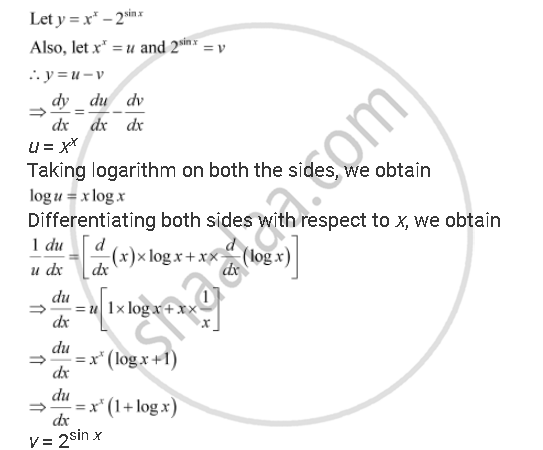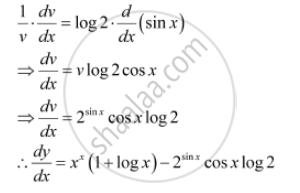Share

# Differentiate the Function with Respect To X. X^X - 2^(Sin X) - CBSE (Science) Class 12 - Mathematics

#### Question

Differentiate the function with respect to x.

x^x - 2^(sin x)

#### SolutionTaking logarithm on both the sides with respect to x, we obtain

log v = sin x - log 2

Differentiating both sides with respect to x, we obtainIs there an error in this question or solution?

#### APPEARS IN

NCERT Solution for Mathematics Textbook for Class 12 (2018 to Current)
Chapter 5: Continuity and Differentiability
Q: 4 | Page no. 178

#### Video TutorialsVIEW ALL 

Solution Differentiate the Function with Respect To X. X^X - 2^(Sin X) Concept: Logarithmic Differentiation.
S